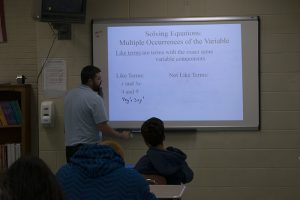# Math Department

Algebra IThis course emphasizes problem-solving techniques and data handling, formulas, linear and non-linear equations, functions and patterns, factoring and quadratic equations, systems of equations, and inequalities. This first-year course is designed for students with some pre-algebra background and a proven math aptitude.

Geometry
The course consists of a more formal investigation of geometric figures and their characteristics to the formulation and proof of formulas and theorems used in mathematics. This course will familiarize students with the basic uses of the spreadsheet on the personal computer. This course is designed for all students who have successfully completed Algebra I.

Algebra II & Trigonometry
This course expands upon the terminology, notation, and problem-solving skills developed in the previous algebra and geometry courses, to include the solving of linear and quadratic equations and inequalities in 2-variables, and the simplifying of fractional and radical expressions. The trigonometric function will be introduced and informally investigated. An effort will also be made to show interrelationships that exist between algebra, geometry, and trigonometry. This course is designed for all students who have successfully completed courses in algebra and geometry.

Advanced Mathematics “A” (Pre-calculus-offered in alternate years)
This course reviews the basic concepts of algebra and geometry, and then examine sequences, series, limits, and related notation. The student will then proceed to the development of the derivative and will apply the derivative to solving maxima-minima problems. This course is designed for all students who have successfully completed geometry and two years of algebra.

Advanced Mathematics “B” (Probability/Statistics/Trigonometry-offered in alternate years)
This course emphasizes probability and statistics and trigonometry. During the first semester, students will study probability and statistics, to include mean, median, standard deviation, correlation, coefficient, etc. Students will prepare a statistical analysis of a topic related to their Senior Project. During the second semester, student will study trigonometry, to include the six trig functions, right triangle applications, Law of Sines, and Law of Cosines, etc. This course is designed for all students who have successfully completed geometry and two years of algebra.